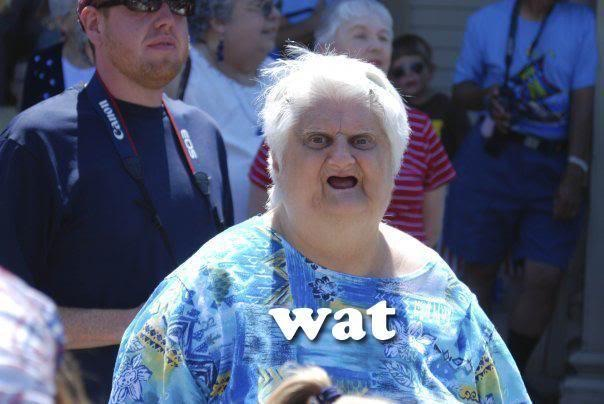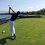# Composite function: help needed

On a recent quiz, I was given the question: If

$f(x)=x^2-2x+1$

$g(x)=ax+b$

$f(g(x))=4x^2-3x-1$

Find a+b.

This first part is not necessary to be read, but it helps for sure. Skip to the $\textbf{(I)}$ if you don't want to read this first half.

Seem simple enough? Let's expand

$(ax+b)^2-2(ax+b)+1=4x^2-3x-1$

$a^2x^2+2abx+b^2-2ax-2b+1=4x^2-3x-1$

Let's match the coefficients of $x^2$

$a^2x^2=4x^2$

$a=\pm2$

Now assume $a=2$ for now, match the coefficients of $x^1$

$4bx-4x=-3x$

$b=\frac{1}{4}$

Yay, it's pretty, therefore $a+b=\frac{9}{4}$

Nope

Match the coefficients of $x^0$ we get

$b^2-2b+1=-1$

$b^2-2b+2=0$

$b=1\pm i$

Wat.

Now here, you might ask, "well what if a=-2" and I will respond, "Glad you asked, but don't worry, I did the calculations and it still doesn't work, I'm just a lazy sloth who is too lazy to type it out." :PBut wait, let's try another approach, because the question doesn't ask for a and b, it just asks for $a+b$. For all we know, $(a,b)=(6+\sqrt i,~\text{Pikachu})$. Also, there are many problems which ask for a+b where you can't solve for a and b.

Here's an example:

A right triangle with one leg 6, hypotenuse a, and other leg b, find $a^2-b^2$

Clearly we can't solve for a and b, but we do know that by Pythagorean theorem, $a^2-b^2=36$

Notice what we did in the original equation, we assumed that$a=\pm 2$ and there is no solution for b. Well, what if a=Charizard???

Now my approach ( I did method (I) on the 40 min quiz which took me about 25 mins to figure out, I still finished the other 5 or 6 questions in time though) was to plug in $x=1$ which im not sure if this is valid. By doing this, $g(1)=a+b$.

Now, we proceed with 3 sub-solutions from here.

$\textbf{(I)}$ Set $f(g(1))=(a+b)^2-2(a+b)-1=4(1)^2-3(1)-1$

$(a+b-1)^2=0$

$a+b=1$

------

Skip to the "skip here" because I think the next two are false

$\textbf{(II)}$. My friend did this and I think its wrong.

Do the reverse of part 1.

$f(g(1))=4(a+b)^2-3(a+b)-1=1^2-2(1)+1$

$(4(a+b)+1)((a+b)-1))=0$

$\therefore a+b=1,-\frac{1}{4}$

$\textbf{(III)}~~$ MY OTHER FRIEND DID THIS, PLEASE CHECK IF THIS IS WRONG, I told him it is but he says it's right. ,Plugging in $g(1)$ into $f(x)$ we get

$f(g(1))=(a+b)^2-2(a+b)+1$

Setting this equal to the $f(g(x))$ we were given yields

$f(g(1))=(a+b)^2-2(a+b)+1=4(a+b)^2-3(a+b)-1$

$0=3(a+b)^2-(a+b)-2$

$0=(3(a+b)+2)((a+b)-1)$

$\therefore a+b=-\frac{2}{3}, ~ 1$

Skip Here

If anyone could provide answers for the following questions I'd greatly appreciate it

What I'm wondering is

1) Why do all the above methods have 1 as a solution? Or is it just a coincidence. Or is it the answer and all others are extraneous.

2) Are the two solutions (2 and 3) false?

3) is this problem unsolvable, false, more than one solution exists, or is it impossible because of some concept like $|x|<0$

4) Am I right?

5) this problem is not Alg 2 difficulty (unless all answers are extraneous)Note by Trevor Arashiro
6 years, 3 months ago

This discussion board is a place to discuss our Daily Challenges and the math and science related to those challenges. Explanations are more than just a solution — they should explain the steps and thinking strategies that you used to obtain the solution. Comments should further the discussion of math and science.

When posting on Brilliant:

• Use the emojis to react to an explanation, whether you're congratulating a job well done , or just really confused .
• Ask specific questions about the challenge or the steps in somebody's explanation. Well-posed questions can add a lot to the discussion, but posting "I don't understand!" doesn't help anyone.
• Try to contribute something new to the discussion, whether it is an extension, generalization or other idea related to the challenge.

MarkdownAppears as
*italics* or _italics_ italics
**bold** or __bold__ bold
- bulleted- list
• bulleted
• list
1. numbered2. list
1. numbered
2. list
Note: you must add a full line of space before and after lists for them to show up correctly
paragraph 1paragraph 2

paragraph 1

paragraph 2

[example link](https://brilliant.org)example link
> This is a quote
This is a quote
    # I indented these lines
# 4 spaces, and now they show
# up as a code block.

print "hello world"
# I indented these lines
# 4 spaces, and now they show
# up as a code block.

print "hello world"
MathAppears as
Remember to wrap math in $$ ... $$ or $ ... $ to ensure proper formatting.
2 \times 3 $2 \times 3$
2^{34} $2^{34}$
a_{i-1} $a_{i-1}$
\frac{2}{3} $\frac{2}{3}$
\sqrt{2} $\sqrt{2}$
\sum_{i=1}^3 $\sum_{i=1}^3$
\sin \theta $\sin \theta$
\boxed{123} $\boxed{123}$

Sort by:

Not every equation needs to have a solution. An extreme example could be like $f(x)=1+x$, find the value of $x$ such that $f(f(x)) = f(x)$.

Solving this would give an absurd situation as $1+(1+x)=1+x \rightarrow 2=1 ?$

- 6 years, 3 months ago

I believe the answer is. "This question is wrong".

There are no corresponding values of $a, b$ which will make the statement true, even if we allow for complex constants.

Staff - 6 years, 3 months ago

The other question that I have is that if I type into wolfram "solve for a and b" the result is false. But if I plug in "find a+b" I get a bunch of ugly results.

- 6 years, 3 months ago

(II) is clearly wrong.

Staff - 6 years, 3 months ago

Thank you for the clarification

- 6 years, 3 months ago

I know that the question didn't specify that a and b are constants, but assume they are.

- 6 years, 3 months ago